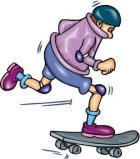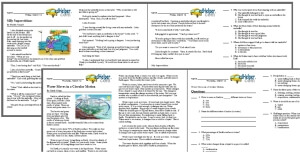Worksheets and No Prep Teaching Resources Reading Comprehension Worksheets Transportation It's Dynamic! Laws of Motion and SpeedTransportation

It's Dynamic! Laws of Motion and Speed
Print It's Dynamic! Laws of Motion and Speed Reading Comprehension with Fifth Grade Work

Print It's Dynamic! Laws of Motion and Speed Reading Comprehension with Sixth Grade Work

Print It's Dynamic! Laws of Motion and Speed Reading Comprehension

Vocabulary
 challenging words: decelerate, dynamics, inertia, instantaneous, inversely, proportional, acceleration, emails, accelerator, velocity, relation, analyze, relationship, conveyor, factor, span content words: Scientist Sir Isaac Newton, First Law, Second Law, Third Law, Disney World

 It's Dynamic! Laws of Motion and Speed By Trista L. Pollard1     What do cars, airplanes, conveyor belts, and emails have in common? They all involve motion. Our daily lives are filled with objects and beings in motion. Each day we expect to see vehicles traveling on the streets and through the air; groceries moving toward cashiers in grocery stores; and emails making their journeys across the wires to their global destinations. As much as we may recognize that motion is an important part of our lives, do we really understand motion?

2     Motion is the change of position of one body in relation to another body. Dynamics is the area of science that focuses on how objects move and the forces that change their motion. To fully understand the motion that is associated with vehicles and machines, you must also understand the scientific laws and principles of motion. It's easy to assume that bodies (beings and objects) are not always moving. However, when scientists analyze the motion of bodies, they do so in reference to other bodies. For example, if we talk about motion in relation to the earth, then all bodies on earth are only moving specific distances at specific points in time. However, if we analyze motion in relation to the sun, then all bodies on earth, including earth itself, are moving continuously. Therefore, our study of motion will focus on movement of bodies in relation to the earth.

3     When bodies move, they travel specific distances in a straight line in specific amounts of time. The rate at which they travel depends on different factors. Those factors are velocity, force, and energy. Let's look at the scientific laws of motion and the principle of velocity. Scientist Sir Isaac Newton (1642-1727) was one of the first scientists to discover the relationship between force and motion. His discoveries helped him to develop three scientific laws of motion. Newton's First Law of Motion states that bodies at rest tend to remain at rest, and that bodies in motion tend to remain in motion. These bodies that are in motion move at a constant speed in a straight line. This is called inertia. The factor that can affect inertia is force. Force, which is the push or pull on an object, can change the motion of an object. Newton's Second Law of Motion involves acceleration and mass. It states that the acceleration of a body is directly proportional to the force applied to that body; and that the mass (weight) of that body is inversely proportional to acceleration. When you increase the force on an object, the object's motion accelerates or increases. If the mass of an object is increased, the motion of the object will decelerate or slow down. Of course if you decrease the object's mass, its motion will accelerate.

Paragraphs 4 to 7:Create Weekly Reading Books Prepare for an entire week at once!